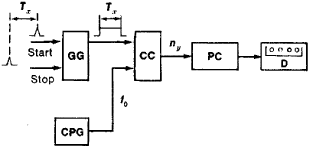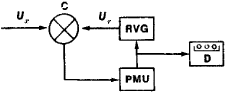# Digital Measuring Device

The following article is from The Great Soviet Encyclopedia (1979). It might be outdated or ideologically biased.

## Digital Measuring Device

a measuring device in which the value of the measured physical quantity is automatically represented by a number on a digital display or by a code, that is, a set of discrete signals.

Digital measuring devices can be divided into digital measuring instruments and digital measuring transducers. Digital measuring instruments are self-contained devices that automatically present the value of the measured quantity on a digital display. Digital measuring transducers lack a digital display; the measurement results are converted into a digital code for subsequent transmission and processing in measuring systems. The most common types of digital measuring devices are those used to measure electrical quantities, such as current, voltage, and frequency. These devices may be used to measure nonelectrical quantities—such as pressure, temperature, speed, and force—if the nonelectrical quantity is first converted into an electrical quantity.

The operation of digital measuring devices is based on the digitization (that is, quantization with respect to level) and coding of the value of the measured physical quantity. The coded signal is fed either to a digital display or to a data transmission and processing system. In a digital display the coded measurement result is converted into a number expressed by numerals, usually in the decimal number system. The most widely used digital displays give two to nine digits. Digital measuring instruments may use electric, cathode-ray, gas-discharge, or liquid-crystal displays. Electric displays may make use of banks of numbered lamps, projection and mosaic systems, multielement lamps, or electroluminiscent cells. Cathode-ray and gas-discharge displays include devices employing multifilament indicating tubes, dekatrons, trochotrons, and numerical indicator tubes. Gas-discharge displays are most widely used because of their simple design, high reliability, and low cost.

The design of digital measuring devices, their accuracy, and their area of application depend on the principle underlying the coding of the measured quantity. The three most commonly used basic principles of analog-to-digital conversion in digital measuring devices are direct conversion, counting, and successive approximation.

In direct conversion, the “memory” of the digital measuring device’s coder contains the set of codes that the device can produce. The value of the measured quantity determines which code is used. This principle is generally employed in devices that measure mechanical displacements.

For example, devices that measure the rotation angle of a shaft typically make use of an encoding disk or drum that is fastened to the shaft. The value of the measured angle is taken from the encoding disk or drum by a reader, which feeds the value, in the form of a coded signal, to the display.

In counter-type devices the quantity being measured is compared with another quantity of the same kind; the second quantity, is obtained through the addition of equal increments. When the two compared quantities become equal, the number of increments is taken as the value of the measured quantity. The measurement error can thus be as great as the value of a single increment.

Such digital measuring devices are used primarily for measuring time intervals, frequency, or other physical quantities (which are first converted into a time interval). Figure 1 provides a schematic diagram of this type of device. The time interval being measured Tx is bounded by the moments at which the two electric pulses “start” and “stop” occur. These pulses control a pulse generator, which produces a gate pulse of duration Tx; the gate pulse is fed to one of the inputs of a coincidence circuit. The other input is connected to a clock pulse generator, which produces pulses at a high repetition frequency f0. The number of pulses ny counted by the counter at the output of the coincidence circuit is equal to f0Tx. If ny/f0Tx, the number ny can be taken as the value of the measured interval. The counter of the clock pulses generates the code corresponding to the numerical value of the interval Tx.Figure 1. Schematic diagram of a digital measuring device used to measure time intervals: (GG) gate pulse generator, (CC) coincidence circuit, (CPG) clock pulse generator, (PC) pulse counter, (D) display, (Tx) time interval being measured, (f0) repetition frequency of clock pulses, (ny) number of pulses in time interval Tx

In digital measuring devices based on successive approximation, as in counter-type devices, the quantity being measured is compared with another quantity of the same kind. In this case, however, the second quantity is obtained by summing increments of unequal magnitude; a given device always makes use of the same set of increments. The sum of the increments of the reference quantity is taken as the numerical value of the measured quantity. The measurement error consequently is not greater than the magnitude of the smallest increment used. The successive-approximation principle is analogous to the method used to weigh an unknown object on a balance: the sum of the known weights balancing the object is taken as the object’s weight. Successive-approximation digital measuring devices are used for electrical quantities, such as voltage, current, and resistance, and for some nonelectrical quantities, which are first converted into electrical quantities.Figure 2. Schematic diagram of a digital DC voltmeter: (C) comparator, (RVG) reference voltage generator, (PMU) programmed memory unit, (D) display, (Ux) voltage being measured, (Ur) reference voltage

Figure 2 presents a schematic diagram of a digital DC voltmeter. The voltage being measured Ux is fed into one of the inputs of the comparator. The reference voltage Ur is applied to the other input from a program-controlled reference voltage generator. The comparator generates one of the two mutually exclusive signals Ur > Ux and UrUx. If the signal UrUx is received, the control memory unit causes the reference voltage generator to increment Ur. If the signal Ur > Ux is received, the control memory unit causes the reference voltage generator to replace the last of the memory increments by a smaller increment. This process is repeated until the increase in Ur becomes equal to the smallest increment possible with the given pulse generator. The control memory unit then generates the code corresponding to the sum of all the increments, and the code is transmitted to the display.

### REFERENCES

Shvetskii, B. I. Elektronnye izmeritel’nye pribory s tsifrovym otschetom, 2nd ed. Kiev, 1970.
Shkurin, G. P. Spravochnik po elektro- i elektronno-izmeritei’nym priboram. Moscow, 1972.
Ornatskii, P. P. Avtomaticheskie izmereniia i pribory, 3rd ed. Kiev, 1973.
Shliandin, V. M. Tsifrovye izmeritel’nye preobrazovateli i pribory. Moscow, 1973.
Elektricheskie izmereniia, 14th ed. Edited by A. V. Fremke. Leningrad, 1973.
Gitis, E. I. Preobrazovateli informatsii dlia elektronnykh tsifrovykh vychislitel’nykh ustroistv, 3rd ed. Moscow, 1975.

N. N. VOSTROKNUTOV